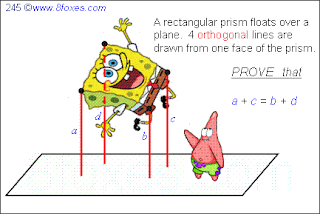Sunday, February 28, 2010

Fox 253 SolutionBleaug goes on:

Energy acquired in free fall from rest position is 1/2.mV2=mg(h0-1/2)

Vertical velocity after bounce is V reduced by a factor equal to cos(2a), where a is the angle of tangent to 1/x at B: Vy = V.cos(2a) and tan(a)=-1/4

Highest point reached after bounce is such that: 1/2.mVy2=mg(h-1/2), so h = 1/2 + cos(2a)2.(h0-1/2)

From simple trigonometry we get cos(2a) = 1-tan(a)2/(1+tan(a)2) = 15/17

So h = 1/2 + (15/17)2 . (h0-1/2) = 1/2 + (225/289). 289/2 = 113.

Friday, February 26, 2010

Fox 254

This is just a model for a very basic structure in Organic Chemistry. Real molecules are more dynamic than this. Atoms vibrate even at very low temperatures.

Wednesday, February 24, 2010

Fox 253

The answer is now confirmed! Will post later...

Tuesday, February 23, 2010

Fox 236 - Solution-1

Let simplicity ring all accross the land...

Monday, February 22, 2010

Fox 252

All along the wall, between us
I see a teacher, there for us
I look at the wall, I see right through it
I lean on the wall, there for us

Sunday, February 21, 2010

Fox 248 - Solution-2

Bleaug states:
Let's take a, b, c to be the sides of A, B, C squares
By construction, the sum of the two shorter sides of D is equal to c. Same result for E.
All right triangles in the picture are similar, so are D and E.
Similar triangles with equal lengths implies D and E are identical (assertion 2)
The two shorter sides of D and E are a and b. So a+b=c (assertion 1)
Triangle D is to F as G is to E, as b is to a. So F/D=E/G=(b/a)^2. Since E=D we get F.G=E^2=D^2 (assertion 3)

Thursday, February 18, 2010

Fox 242 - Counterexample-2

Yu claims:
A rectangle is a trapezoid. The shaded area (2 equal triangles) = ¼ of the rectangle.The above trapezoid has the same area as the rectangle above. As P approaches Q, the height of the upper shaded triangle increases faster than the height of the lower shaded triangle decreases. .: the shaded area > ¼ of the trapezoid.

Fox 248

Never delay a good idea.
Once you let it go,
it may never have a chance to resurrect.
--- Himalayan Fox

Monday, February 15, 2010

Fox 245http://www.8foxes.com/
Ignore the curvatures, SpongeBob gets wobbly when he's overjoyed.
By the way, SpongeBob and Patrick are owned by Viacom International, Inc.
Freedom to the Bikini-Bottom!

Saturday, February 13, 2010

Fox 243 - Solution

Bleaug claims the following:
Almost a pure geometric proof this time (only requires Pythagorean theorem).
- TIJ is the section of the pyramid by a plane orthogonal to base and parallel to BC and AD
- by construction, TI is orthogonal to AB, and TJ to CD
- hence: AT^2-BT^2 = AI^2+IT^2-BI^2-IT^2 = AI^2-BI^2, similarly DT^2-CT^2 = DJ^2-CJ^2
- since AIB is a translation of DJC: AT^2-BT^2 = DT^2-CT^2
- from which we get DT^2 = AT^2-BT^2+CT^2 = 9-2+1 = 8

Friday, February 12, 2010

Fox 244

Look into thy heart, and see,
it desireth to fly in Heavens,
whilst there are knots pulling thee
to cruelly darketh corners!
--- Dervish Fox

Wednesday, February 10, 2010

Fox 242 - Counterexamplehttp://www.8foxes.com/
Bleaug has submitted this counterexample. He smells right :)

Easiest way is to get a counter-example:
assume the upper side of the trapezoid collapses to a single point,
the pink area corresponds to 1/4 of the trapezoid/triangle area.
The (non-empty) blue area is in excess.

Friday, February 5, 2010

Fox 241

YES, the solution has NOW been confirmed!!
We will post these excellent solutions here later!
There are at least one more slick solution, not identified yet!
Thank you guys for the great work!

Wednesday, February 3, 2010

Fox 240

Now this asks little more than geometry.
Note that the random process described in the question is discrete.
Ladybugs "appear" in next vertices at the same time.

Tuesday, February 2, 2010

Fox 239

Make things as simple as possible, but not simpler.